# Programming for Geographical Information Analysis Core Skills Modules

• Slides: 55Programming for Geographical Information Analysis: Core Skills Modules and PackagesReview We've seen that a module is a file that can contain classes as well as its own variables. We've seen that you need to import it to access the code, and then use the module name to refer to it. import module 1 a = module 1. Class. Name()This lecture Import. Modules. Packages. Useful standard library packages. Useful external packages.Packages modules: usually single files to do some set of jobs packages: modules with a namespace, that is, a unique way of referring to them libraries: a generic name for a collection of code you can use to get specific types of job done.Packages The Standard Python Library comes with a number of other packages which are not imported automatically. We need to import them to use them.Import import agentframework point_1 = agentframework. Agent() This is a very explicit style. There is little ambiguity about which Agent we are after (if other imported modules have Agent classes). This is safest as you have to be explicit about the module. Provided there aren't two modules with the same name and class, you are fine. If you're sure there are no other Agent, you can: from agentframework import Agent point_1 = Agent() This just imports this one class.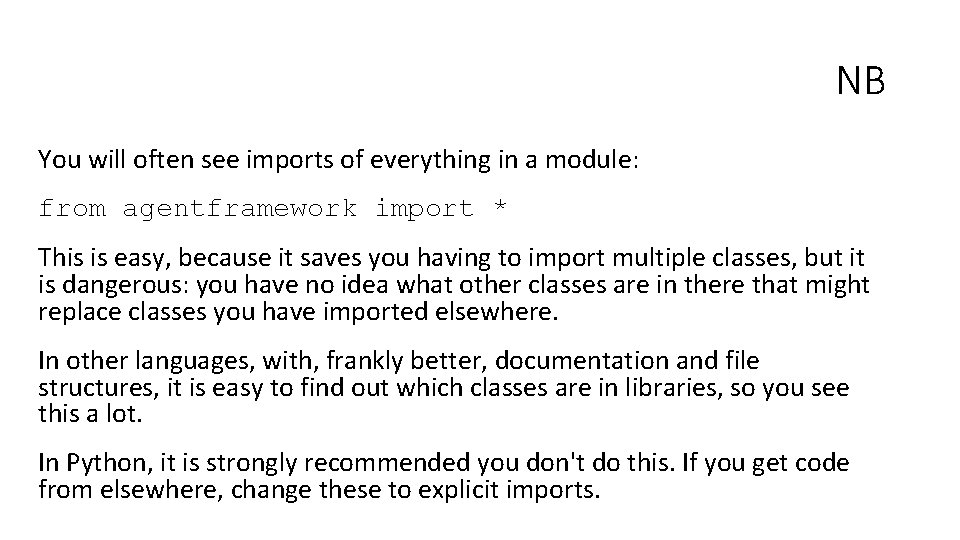NB You will often see imports of everything in a module: from agentframework import * This is easy, because it saves you having to import multiple classes, but it is dangerous: you have no idea what other classes are in there that might replace classes you have imported elsewhere. In other languages, with, frankly better, documentation and file structures, it is easy to find out which classes are in libraries, so you see this a lot. In Python, it is strongly recommended you don't do this. If you get code from elsewhere, change these to explicit imports.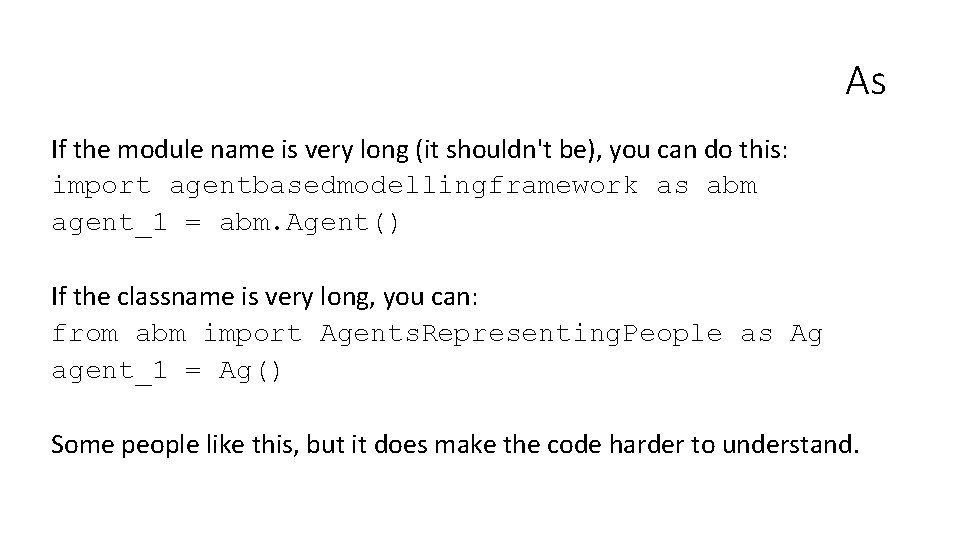As If the module name is very long (it shouldn't be), you can do this: import agentbasedmodellingframework as abm agent_1 = abm. Agent() If the classname is very long, you can: from abm import Agents. Representing. People as Ag agent_1 = Ag() Some people like this, but it does make the code harder to understand.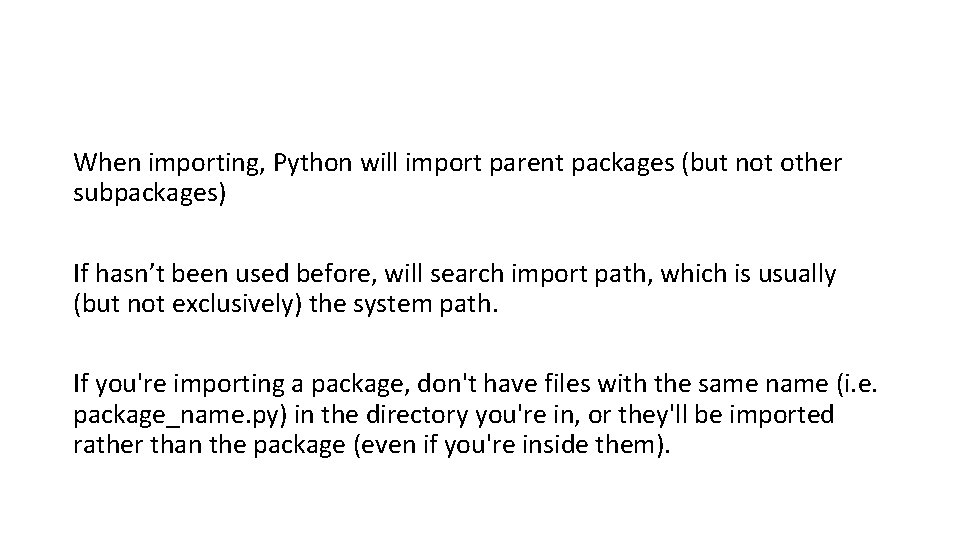When importing, Python will import parent packages (but not other subpackages) If hasn’t been used before, will search import path, which is usually (but not exclusively) the system path. If you're importing a package, don't have files with the same name (i. e. package_name. py) in the directory you're in, or they'll be imported rather than the package (even if you're inside them).Interpreter To reload a module: importlib. reload(modulename) In Spyder, just re-run the module file. Remember to do this if you update it.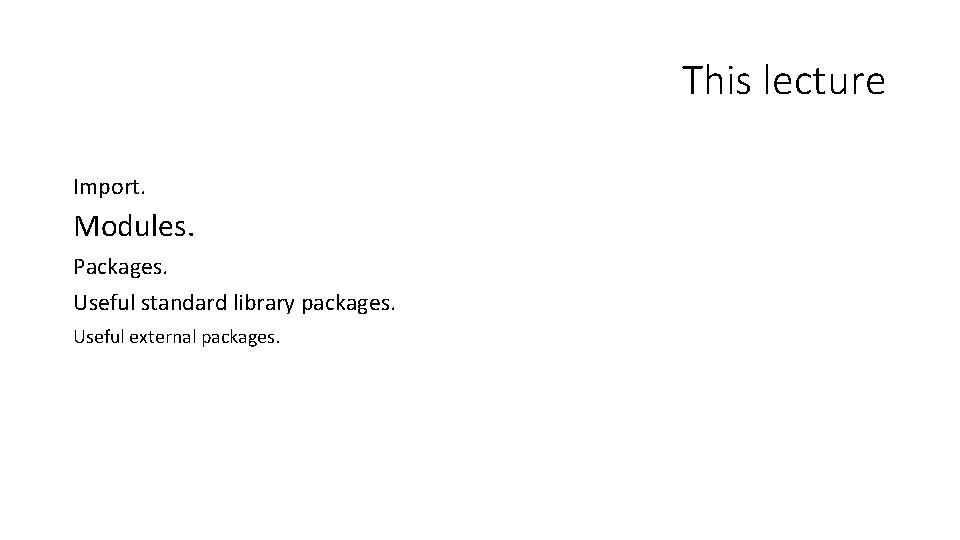This lecture Import. Modules. Packages. Useful standard library packages. Useful external packages.Modules and Packages Modules are single files that can contain multiple classes, variables, and functions. The main difference when thinking of module and scripts is that the former is generally imported, and the latter generally runs directly. Packages are collections of modules structured using a directory tree.Running module code Although we've concentrated on classes, you can import and run module-level functions, and access variables. import module 1 print(module 1. module_variable) module 1. module_function() a = module 1. Class. Name()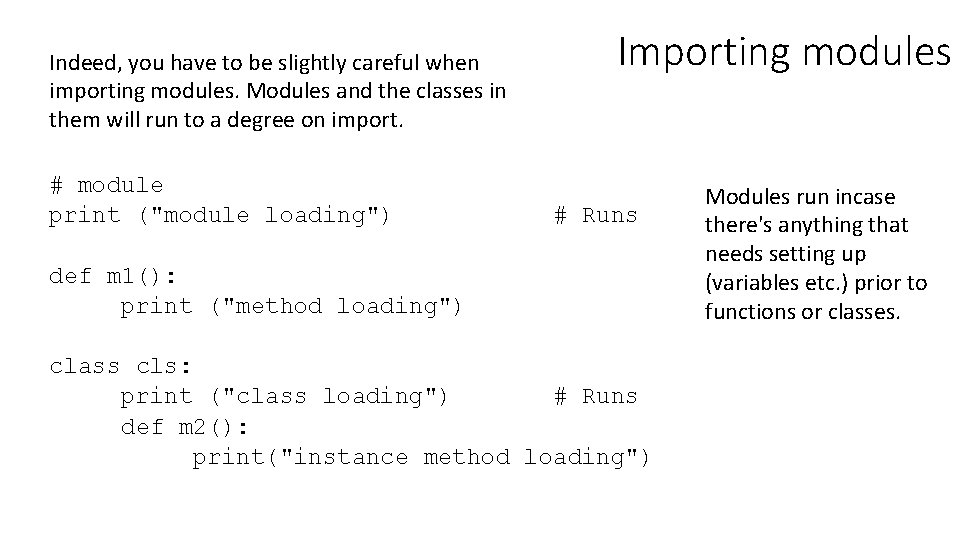Indeed, you have to be slightly careful when importing modules. Modules and the classes in them will run to a degree on import. # module print ("module loading") Importing modules # Runs def m 1(): print ("method loading") class cls: print ("class loading") # Runs def m 2(): print("instance method loading") Modules run incase there's anything that needs setting up (variables etc. ) prior to functions or classes.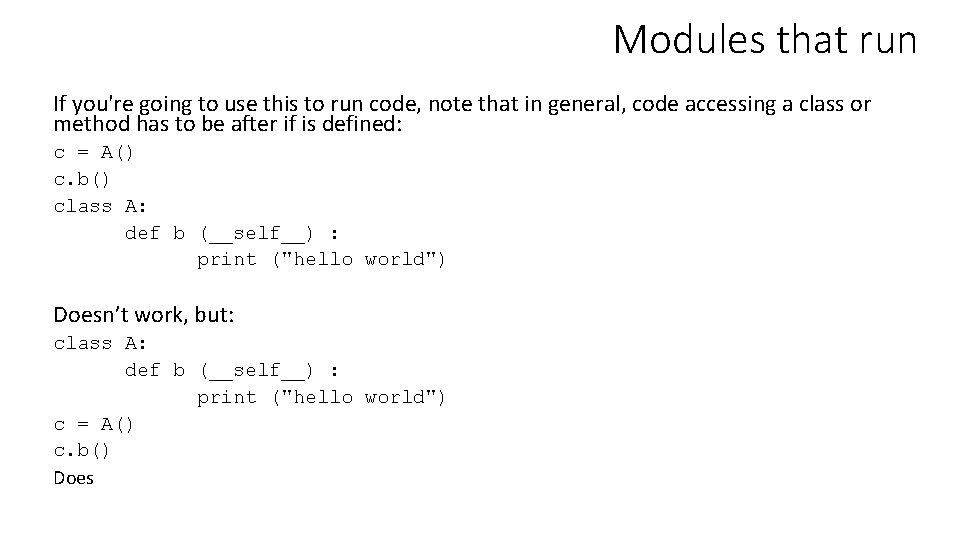Modules that run If you're going to use this to run code, note that in general, code accessing a class or method has to be after if is defined: c = A() c. b() class A: def b (__self__) : print ("hello world") Doesn’t work, but: class A: def b (__self__) : print ("hello world") c = A() c. b() Does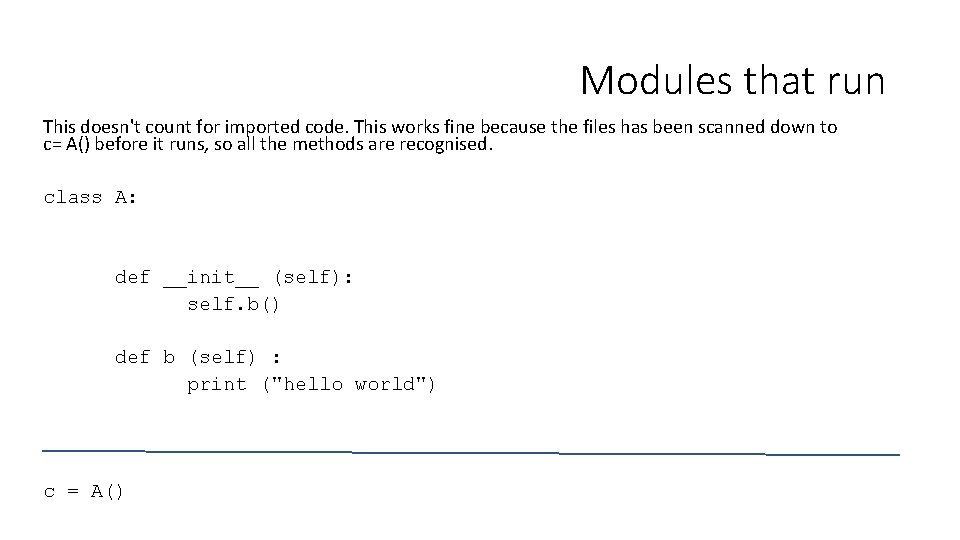Modules that run This doesn't count for imported code. This works fine because the files has been scanned down to c= A() before it runs, so all the methods are recognised. class A: def __init__ (self): self. b() def b (self) : print ("hello world") c = A()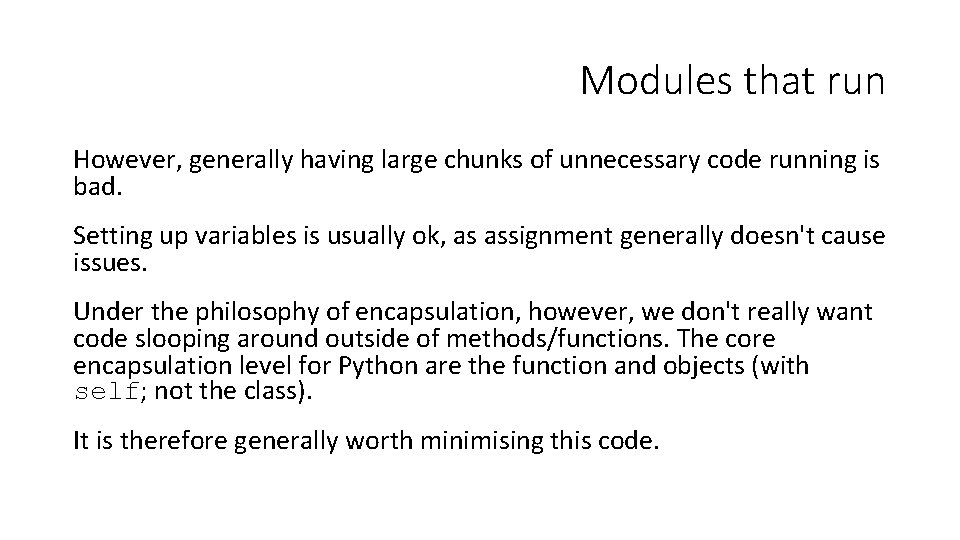Modules that run However, generally having large chunks of unnecessary code running is bad. Setting up variables is usually ok, as assignment generally doesn't cause issues. Under the philosophy of encapsulation, however, we don't really want code slooping around outside of methods/functions. The core encapsulation level for Python are the function and objects (with self; not the class). It is therefore generally worth minimising this code.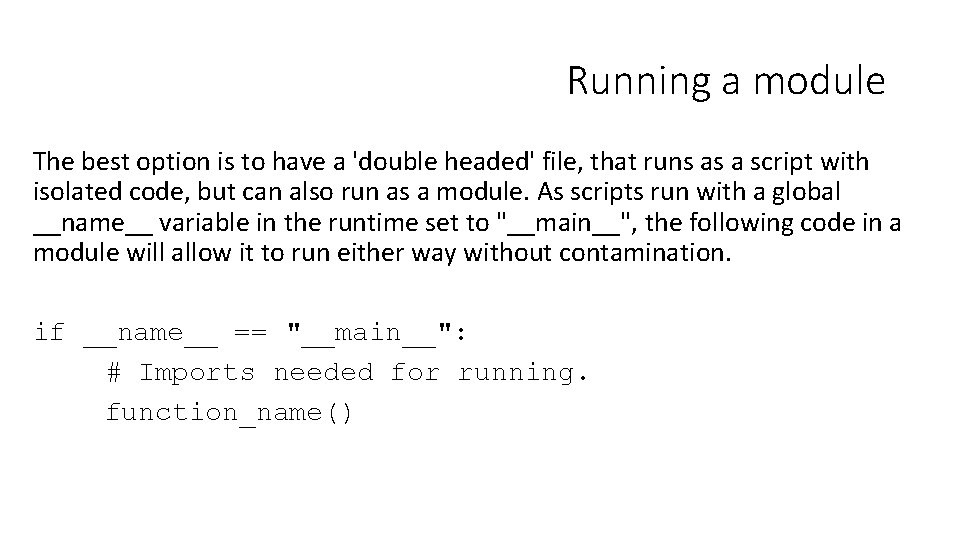Running a module The best option is to have a 'double headed' file, that runs as a script with isolated code, but can also run as a module. As scripts run with a global __name__ variable in the runtime set to "__main__", the following code in a module will allow it to run either way without contamination. if __name__ == "__main__": # Imports needed for running. function_name()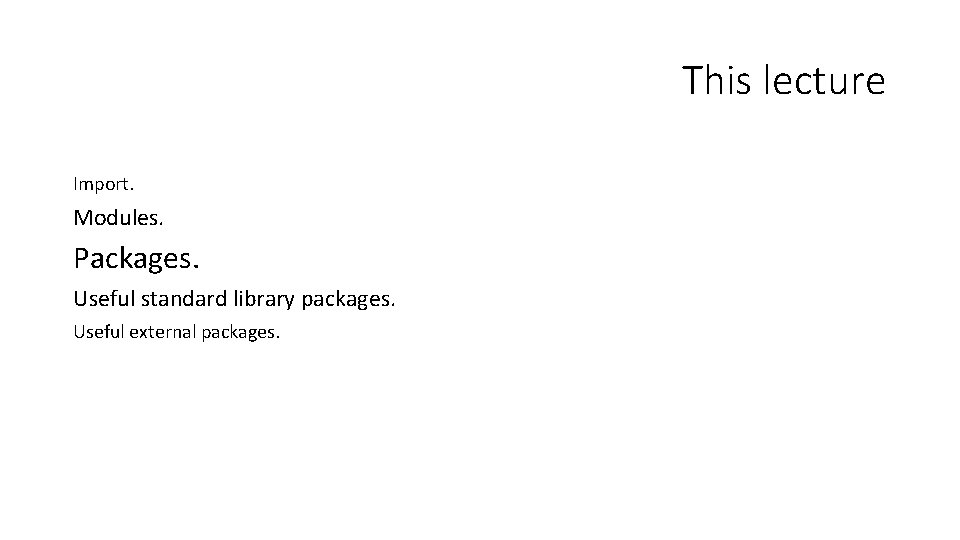This lecture Import. Modules. Packages. Useful standard library packages. Useful external packages.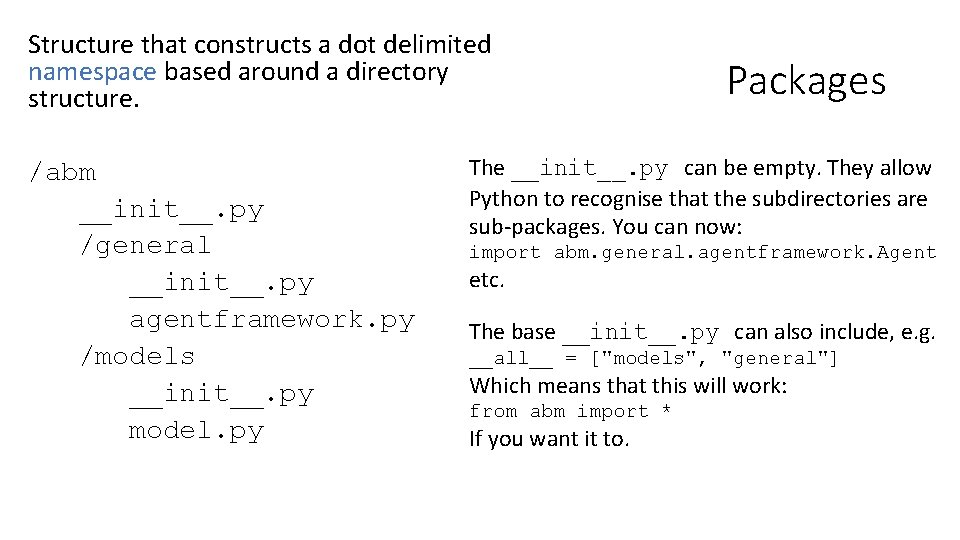Structure that constructs a dot delimited namespace based around a directory structure. /abm __init__. py /general __init__. py agentframework. py /models __init__. py model. py Packages The __init__. py can be empty. They allow Python to recognise that the subdirectories are sub-packages. You can now: import abm. general. agentframework. Agent etc. The base __init__. py can also include, e. g. __all__ = ["models", "general"] Which means that this will work: from abm import * If you want it to.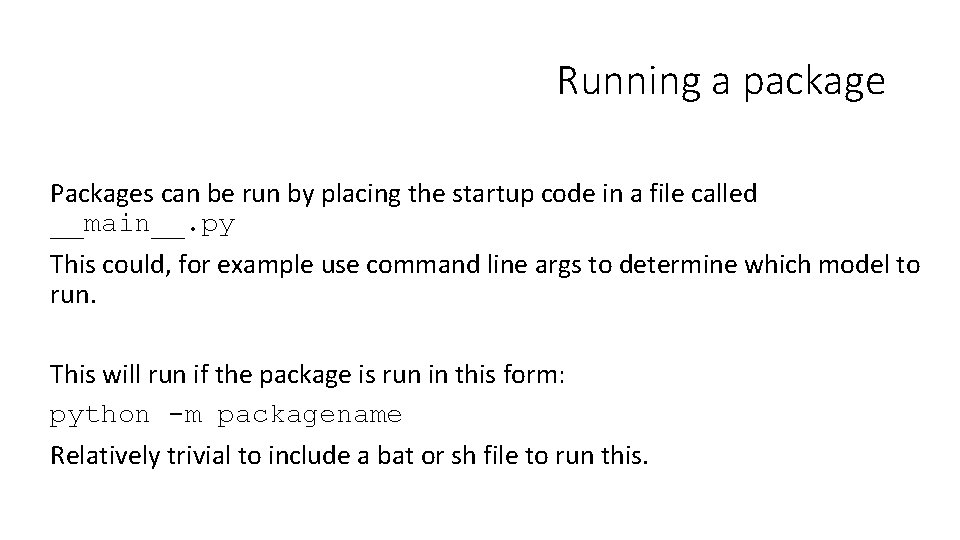Running a package Packages can be run by placing the startup code in a file called __main__. py This could, for example use command line args to determine which model to run. This will run if the package is run in this form: python -m packagename Relatively trivial to include a bat or sh file to run this.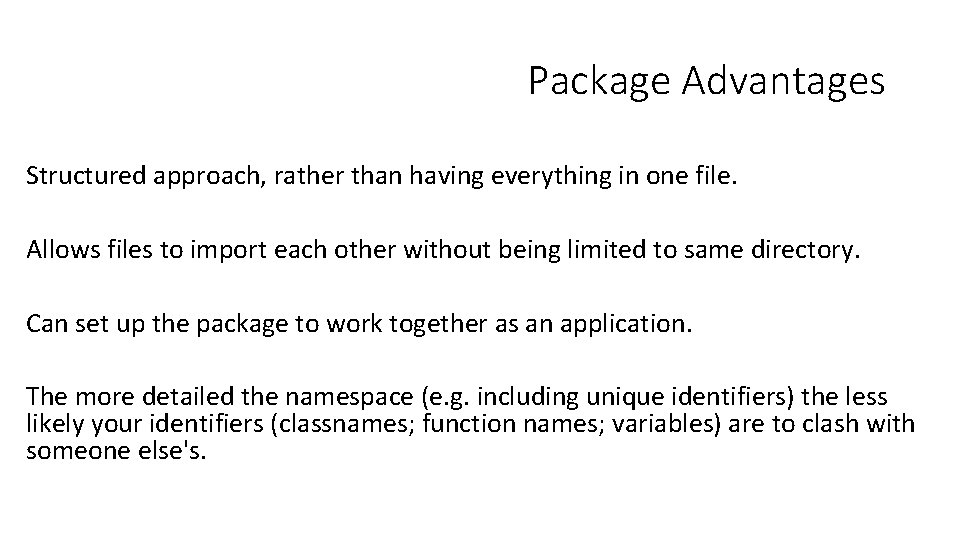Package Advantages Structured approach, rather than having everything in one file. Allows files to import each other without being limited to same directory. Can set up the package to work together as an application. The more detailed the namespace (e. g. including unique identifiers) the less likely your identifiers (classnames; function names; variables) are to clash with someone else's.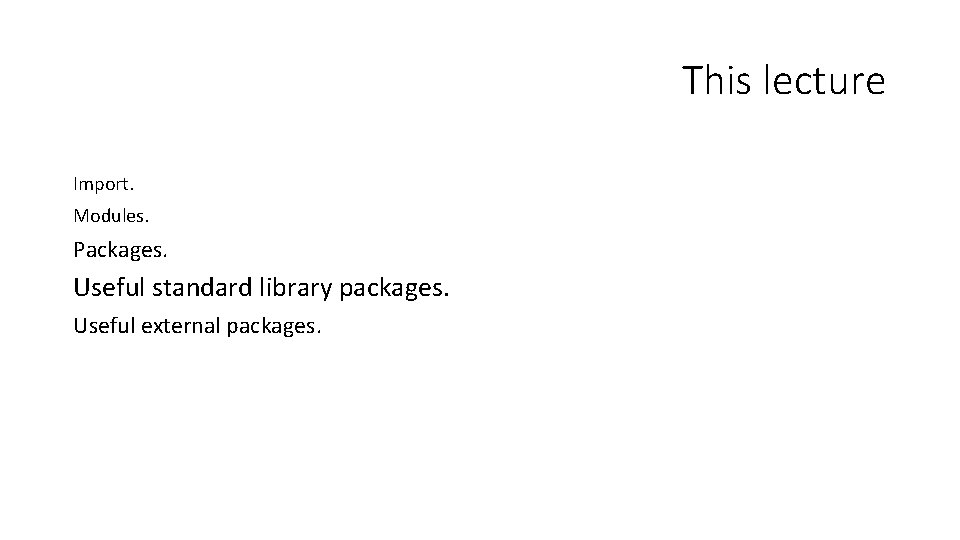This lecture Import. Modules. Packages. Useful standard library packages. Useful external packages.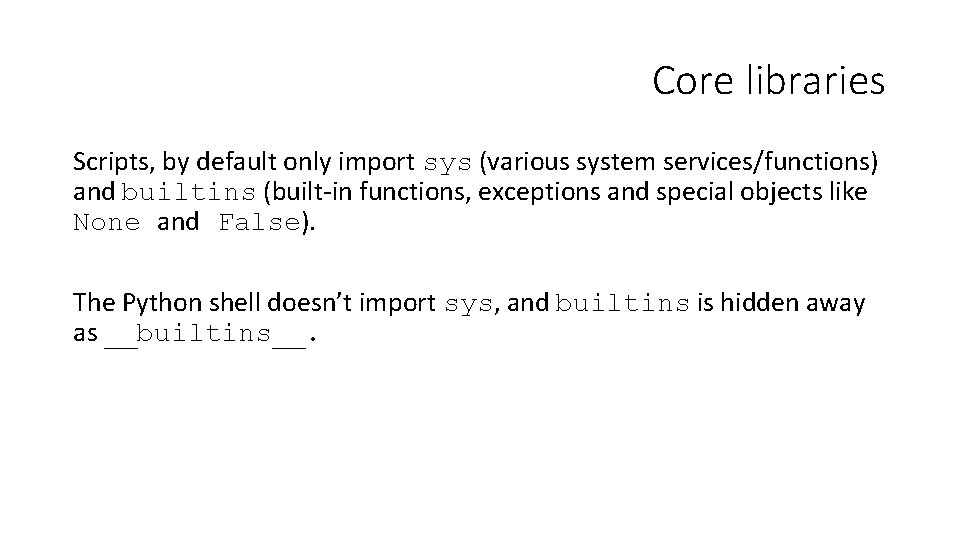Core libraries Scripts, by default only import sys (various system services/functions) and builtins (built-in functions, exceptions and special objects like None and False). The Python shell doesn’t import sys, and builtins is hidden away as __builtins__.Built in functions abs() dict() help() min() setattr() all() dir() hex() next() slice() any() divmod() id() object() sorted() ascii() enumerate() input() oct() staticmethod() bin() eval() int() open() str() bool() exec() isinstance() ord() sum() bytearray() filter() issubclass() pow() super() bytes() float() iter() print() tuple() callable() format() len() property() type() chr() frozenset() list() range() vars() classmethod() getattr() locals() repr() zip() compile() globals() map() reversed() __import__() complex() hasattr() max() round() delattr() hash() memoryview() set() https: //docs. python. org/3/library/functions. html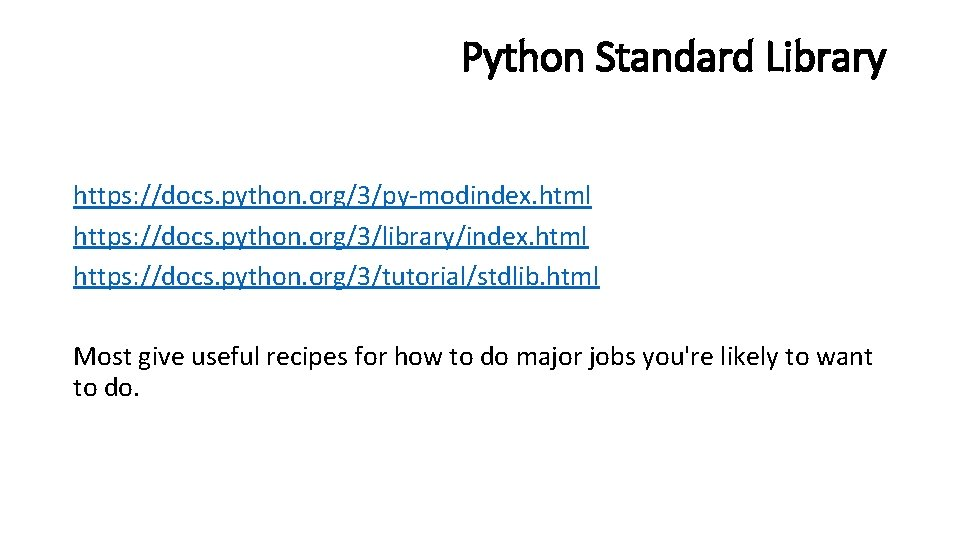Python Standard Library https: //docs. python. org/3/py-modindex. html https: //docs. python. org/3/library/index. html https: //docs. python. org/3/tutorial/stdlib. html Most give useful recipes for how to do major jobs you're likely to want to do.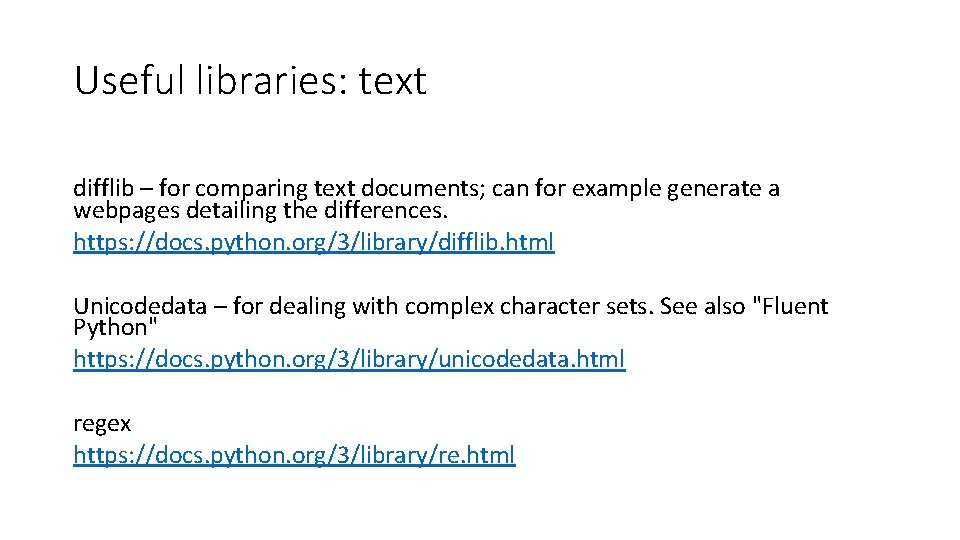Useful libraries: text difflib – for comparing text documents; can for example generate a webpages detailing the differences. https: //docs. python. org/3/library/difflib. html Unicodedata – for dealing with complex character sets. See also "Fluent Python" https: //docs. python. org/3/library/unicodedata. html regex https: //docs. python. org/3/library/re. html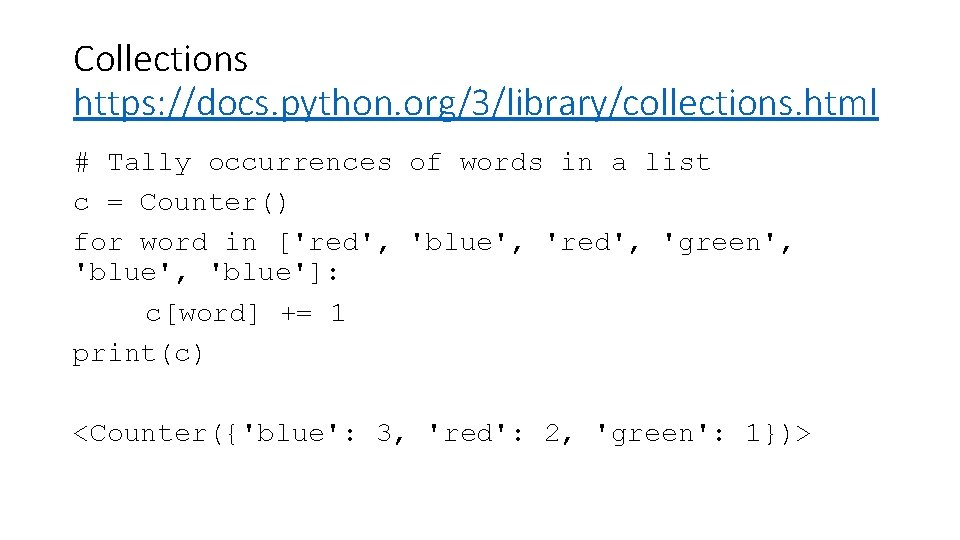Collections https: //docs. python. org/3/library/collections. html # Tally occurrences of words in a list c = Counter() for word in ['red', 'blue', 'red', 'green', 'blue']: c[word] += 1 print(c) <Counter({'blue': 3, 'red': 2, 'green': 1})>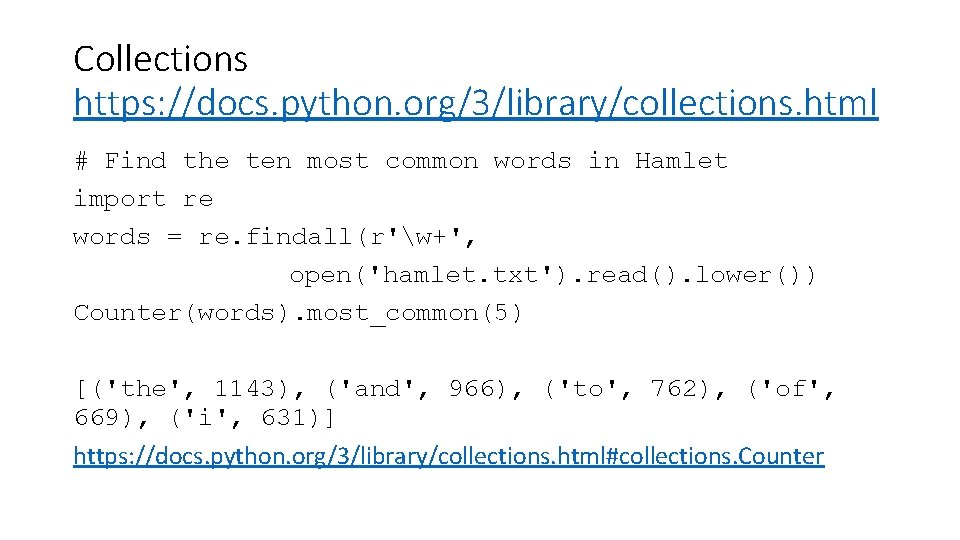Collections https: //docs. python. org/3/library/collections. html # Find the ten most common words in Hamlet import re words = re. findall(r'w+', open('hamlet. txt'). read(). lower()) Counter(words). most_common(5) [('the', 1143), ('and', 966), ('to', 762), ('of', 669), ('i', 631)] https: //docs. python. org/3/library/collections. html#collections. Counter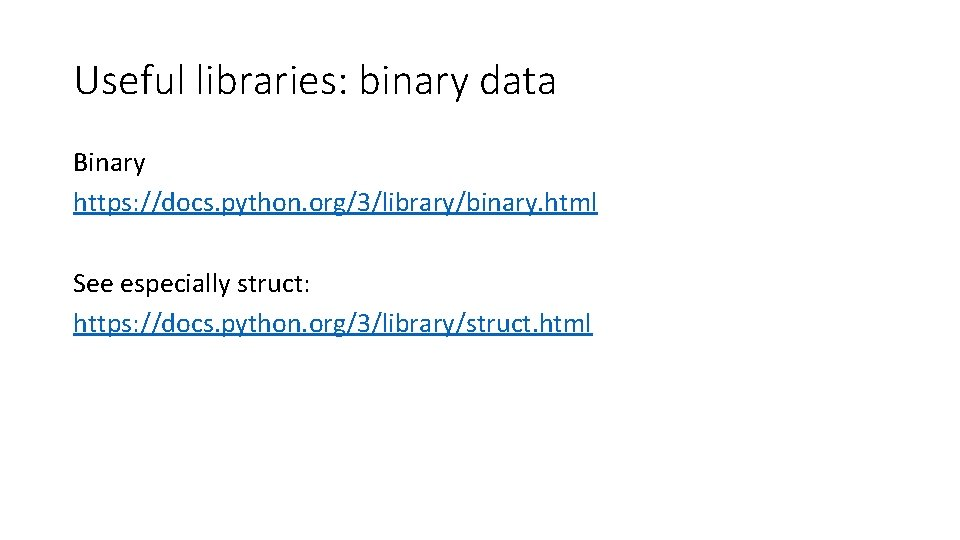Useful libraries: binary data Binary https: //docs. python. org/3/library/binary. html See especially struct: https: //docs. python. org/3/library/struct. htmlUseful libraries: maths math https: //docs. python. org/3/library/math. html decimal — Does for floating points what ints do; makes them exact https: //docs. python. org/3/library/decimal. html fractions — Rational numbers (For dealing with numbers as fractions https: //docs. python. org/3/library/fractions. htmlStatistics https: //docs. python. org/3/library/statistics. html mean() Arithmetic mean (“average”) of data. harmonic_mean() Harmonic mean of data. median() Median (middle value) of data. median_low() Low median of data. median_high() High median of data. median_grouped() Median, or 50 th percentile, of grouped data. mode() Mode (most common value) of discrete data. pstdev() Population standard deviation of data. pvariance() Population variance of data. stdev() Sample standard deviation of data. variance() Sample variance of data.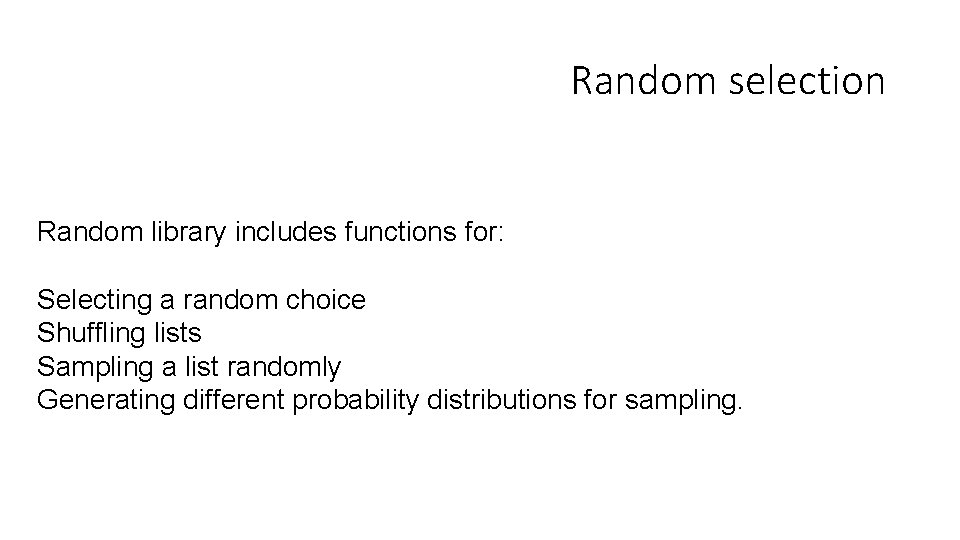Random selection Random library includes functions for: Selecting a random choice Shuffling lists Sampling a list randomly Generating different probability distributions for sampling.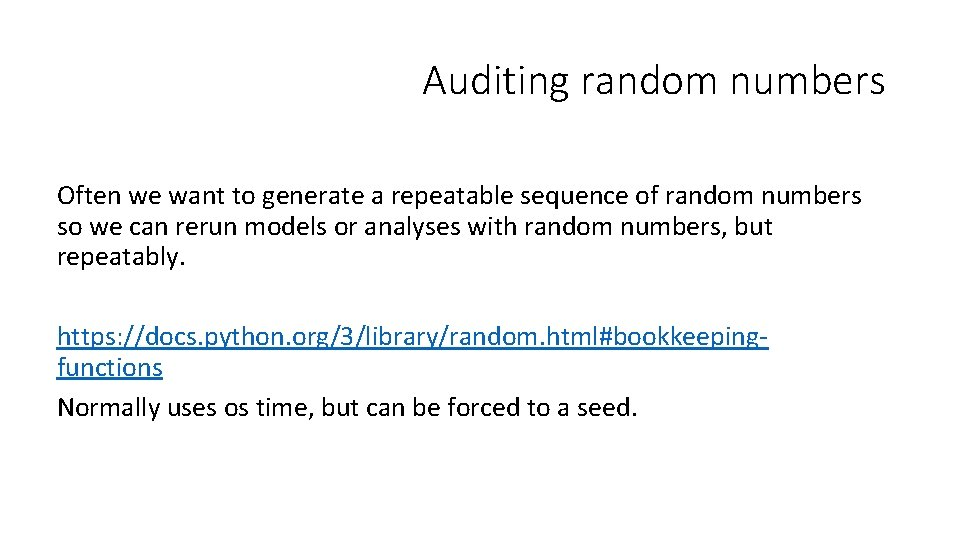Auditing random numbers Often we want to generate a repeatable sequence of random numbers so we can rerun models or analyses with random numbers, but repeatably. https: //docs. python. org/3/library/random. html#bookkeepingfunctions Normally uses os time, but can be forced to a seed.Useful libraries: lists/arrays bisect — Array bisection algorithm (efficient large sorted arrays for finding stuff) https: //docs. python. org/3/library/bisect. html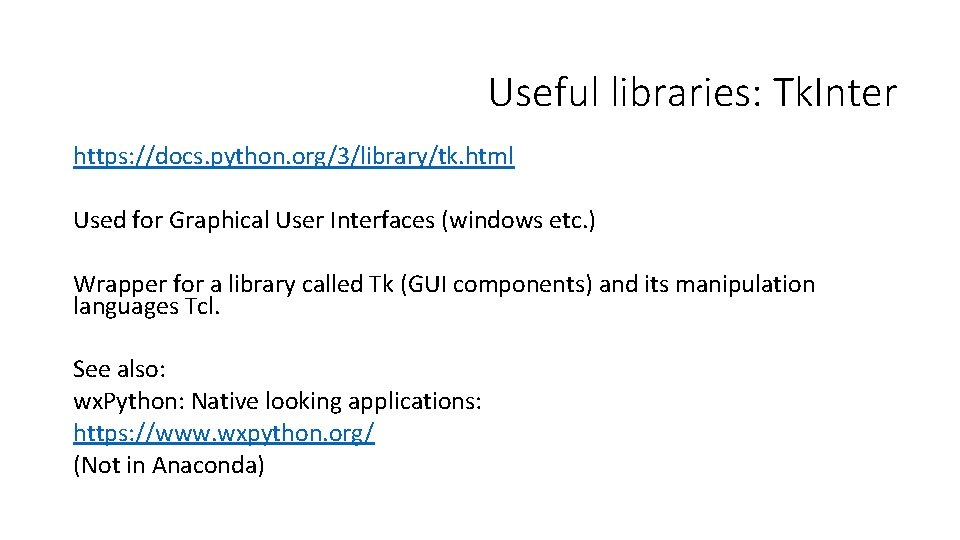Useful libraries: Tk. Inter https: //docs. python. org/3/library/tk. html Used for Graphical User Interfaces (windows etc. ) Wrapper for a library called Tk (GUI components) and its manipulation languages Tcl. See also: wx. Python: Native looking applications: https: //www. wxpython. org/ (Not in Anaconda)Turtle https: //docs. python. org/3/library/turtle. html For drawing shapes. TKInter will allow you to load and display images, but there additional external libraries better set up for this, including Pillow: http: //python-pillow. org/Useful libraries: talking to the outside world Serial ports https: //docs. python. org/3/faq/library. html#how-do-i-access-the-serial-rs 232 -port argparse — Parser for command-line options, arguments and sub-commands https: //docs. python. org/3/library/argparse. html datetime https: //docs. python. org/3/library/datetime. html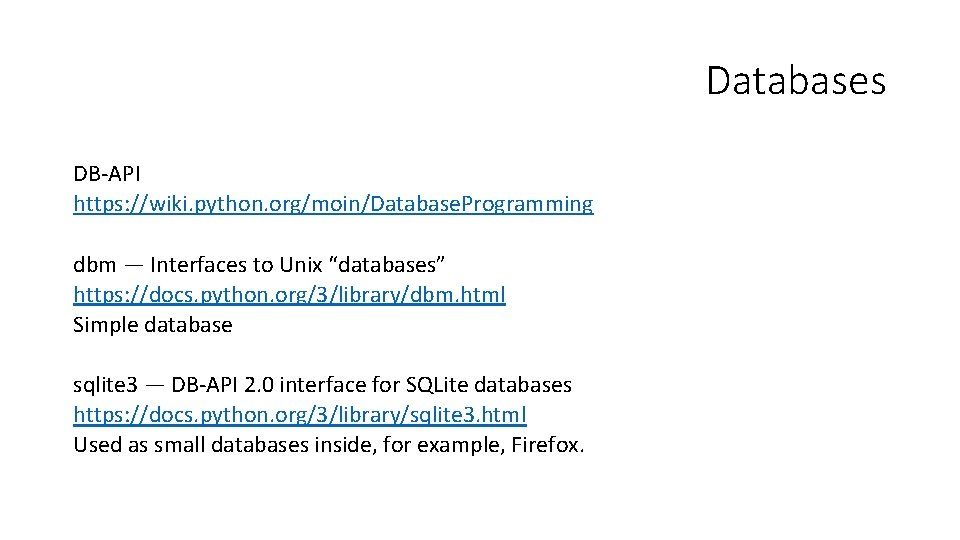Databases DB-API https: //wiki. python. org/moin/Database. Programming dbm — Interfaces to Unix “databases” https: //docs. python. org/3/library/dbm. html Simple database sqlite 3 — DB-API 2. 0 interface for SQLite databases https: //docs. python. org/3/library/sqlite 3. html Used as small databases inside, for example, Firefox.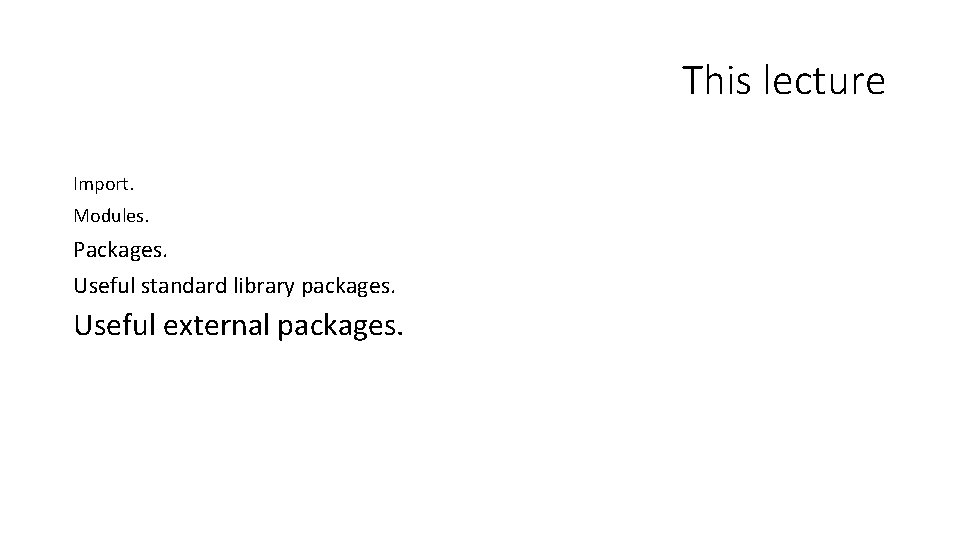This lecture Import. Modules. Packages. Useful standard library packages. Useful external packages.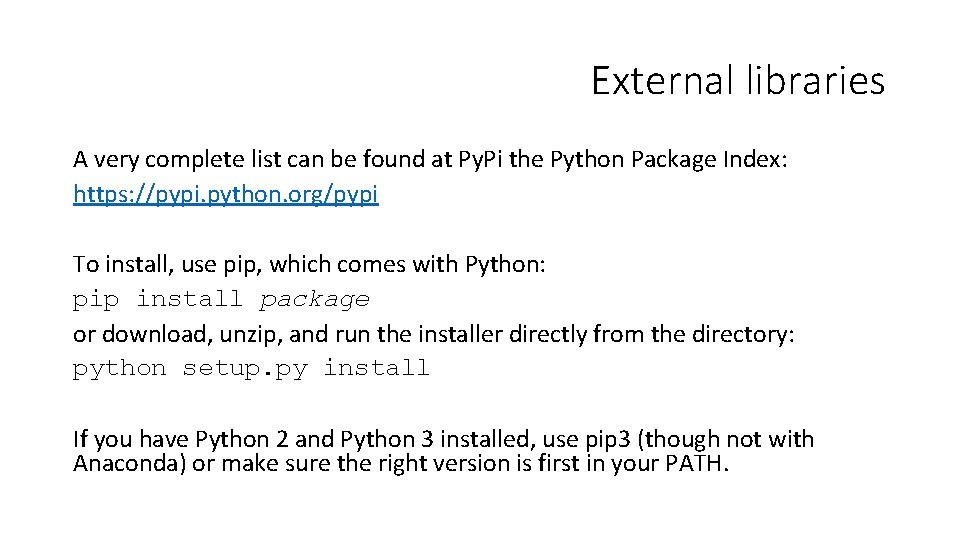External libraries A very complete list can be found at Py. Pi the Python Package Index: https: //pypi. python. org/pypi To install, use pip, which comes with Python: pip install package or download, unzip, and run the installer directly from the directory: python setup. py install If you have Python 2 and Python 3 installed, use pip 3 (though not with Anaconda) or make sure the right version is first in your PATH.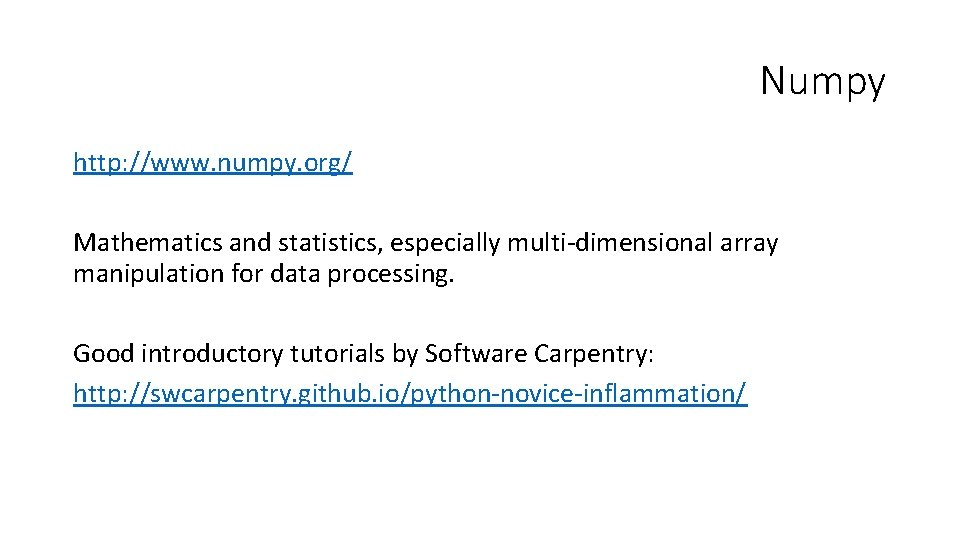Numpy http: //www. numpy. org/ Mathematics and statistics, especially multi-dimensional array manipulation for data processing. Good introductory tutorials by Software Carpentry: http: //swcarpentry. github. io/python-novice-inflammation/Numpy data Perhaps the nicest thing about numpy is its handling of complicated 2 D datasets. It has its own array types which overload the indexing operators. Note the difference in the below from the standard [1 d][2 d] notation: import numpy data = numpy. int_([ [1, 2, 3, 4, 5], [10, 20, 30, 40, 50], [100, 200, 300, 400, 500] ]) print(data[0, 0]) print(data[1: 3, 1: 3]) # # 1 [[20 30][200 300]] On a standard list, data[1: 3] wouldn't work, at best data[1: 3][1: 3] would give you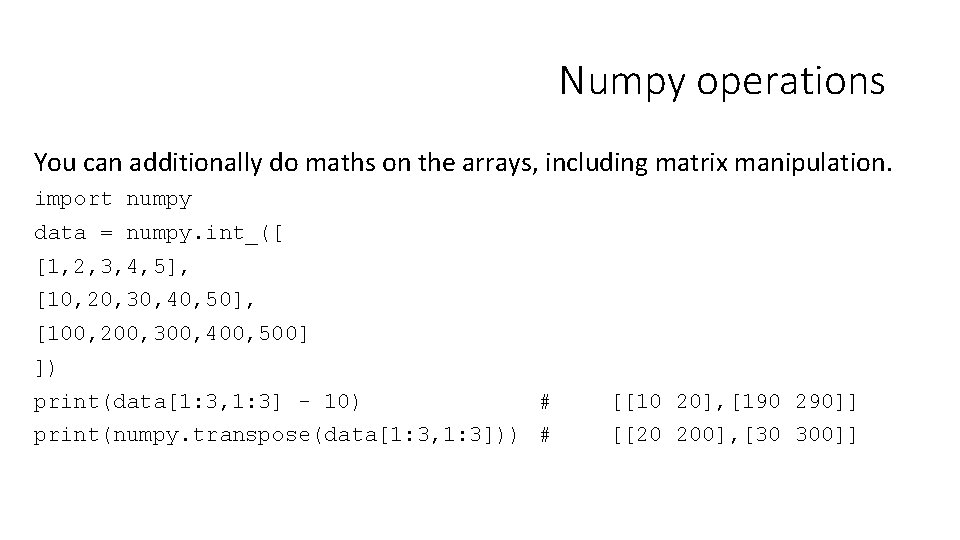Numpy operations You can additionally do maths on the arrays, including matrix manipulation. import numpy data = numpy. int_([ [1, 2, 3, 4, 5], [10, 20, 30, 40, 50], [100, 200, 300, 400, 500] ]) print(data[1: 3, 1: 3] - 10) # [[10 20], [190 290]] print(numpy. transpose(data[1: 3, 1: 3])) # [[20 200], [30 300]]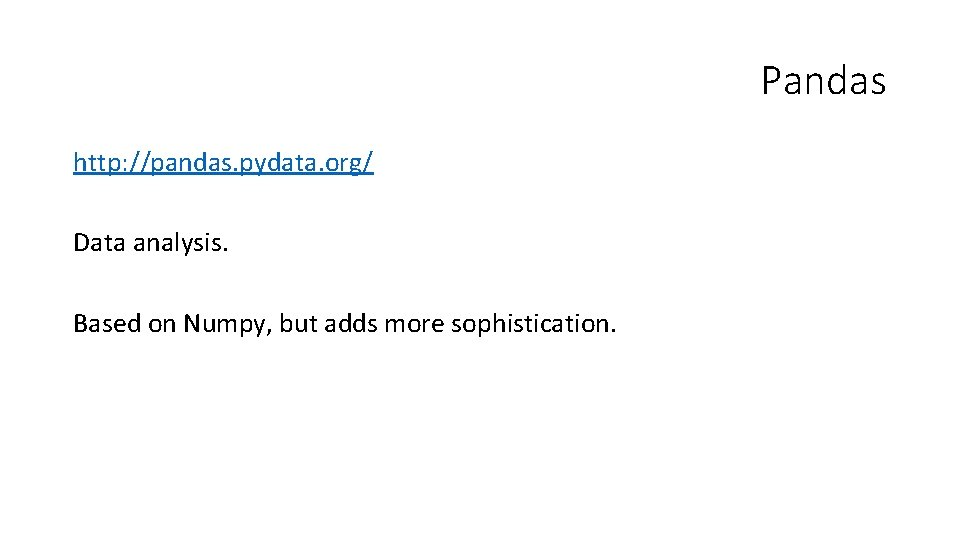Pandas http: //pandas. pydata. org/ Data analysis. Based on Numpy, but adds more sophistication.Pandas data focuses around Data. Frames, 2 D arrays with addition abilities to name and use rows and columns. import pandas df = pandas. Data. Frame( data, # numpy array from before. index=['i', 'iii'], columns=['A', 'B', 'C', 'D', 'E'] ) print (data['A']) print(df. mean(0)['A']) print(df. mean(1)['i']) Prints: i 1 ii 10 iii 100 Name: A, dtype: int 32 37. 0 3. 0 Pandas data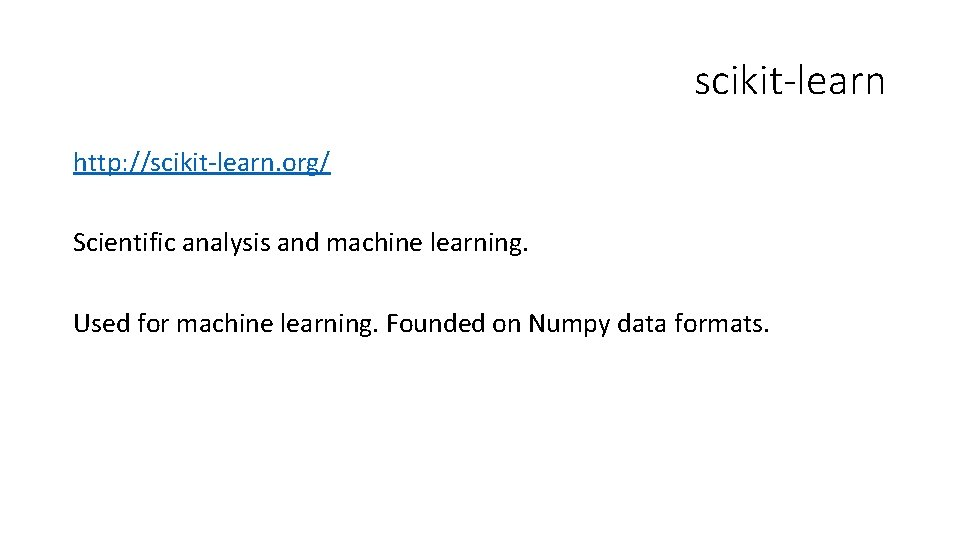scikit-learn http: //scikit-learn. org/ Scientific analysis and machine learning. Used for machine learning. Founded on Numpy data formats.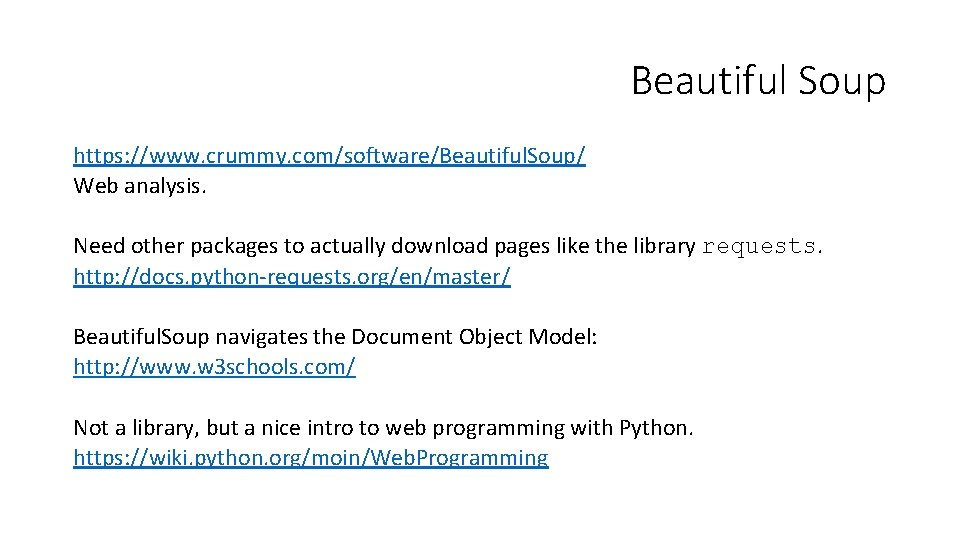Beautiful Soup https: //www. crummy. com/software/Beautiful. Soup/ Web analysis. Need other packages to actually download pages like the library requests. http: //docs. python-requests. org/en/master/ Beautiful. Soup navigates the Document Object Model: http: //www. w 3 schools. com/ Not a library, but a nice intro to web programming with Python. https: //wiki. python. org/moin/Web. ProgrammingTweepy http: //www. tweepy. org/ Downloading Tweets for analysis. You'll also need a developer key: http: //themepacific. com/how-to-generate-api-key-consumer-tokenaccess-key-for-twitter-oauth/994/ Most social media sites have equivalent APIs (functions to access them) and modules to use those.NLTK http: //www. nltk. org/ Natural Language Toolkit. Parse text and analyse everything from Parts Of Speech to positivity or negativity of statements (sentiment analysis).Celery http: //www. celeryproject. org/ Concurrent computing / parallelisation. For splitting up programs and running them on multiple computers e. g. to remove memory limits. See also: https: //docs. python. org/3/library/concurrency. html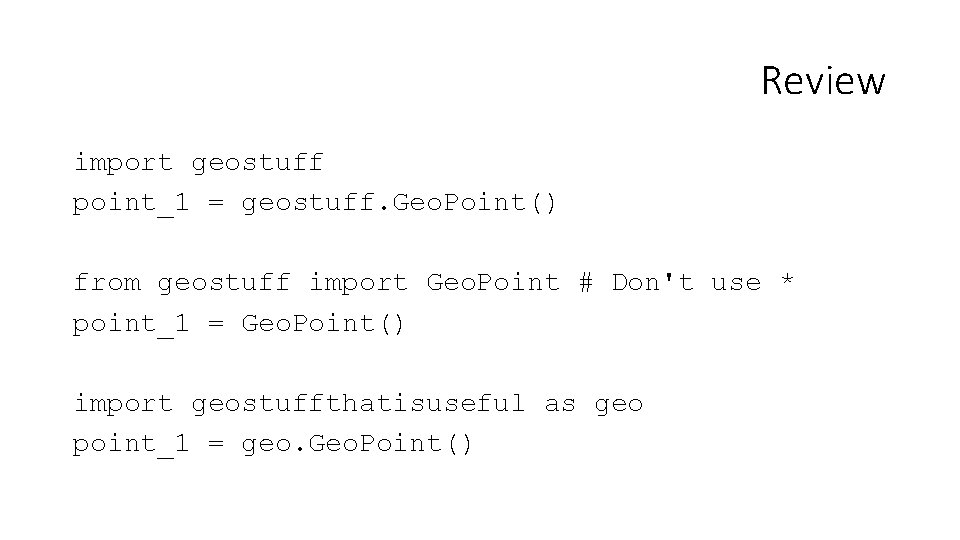Review import geostuff point_1 = geostuff. Geo. Point() from geostuff import Geo. Point # Don't use * point_1 = Geo. Point() import geostuffthatisuseful as geo point_1 = geo. Geo. Point()Review Generally, on import, loose code in modules and classes will run. Avoid this by placing all code in functions and use the following to isolate code to run if you want the module to also run as a script: if __name__ == "__main__": # Imports needed for running. function_name()Review In general in scripts and modules code has to be defined before it can be used within the same module. class A: def b (__self__) : print ("hello world") c = A() c. b()Key standard libraries to study: builtins/pathlib/os math/statistics decimal/fraction regex datetime Key external libraries to study: matplotlib numpy pandas beautifulsoup tkinter Review